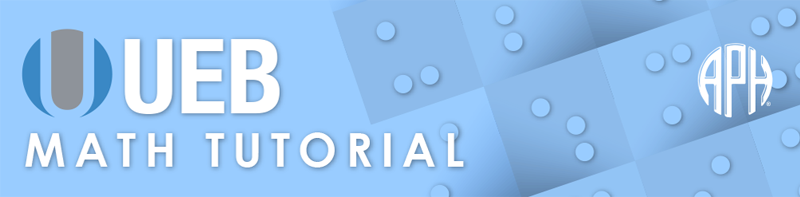Practice Problems -   -  Use 6 Dot Entry   Switch to Nemeth Tutorial

# Lesson 5.4: Segments, Lines, and Rays

## Symbols

$\text{bar over previous item}$

$\text{simple right-pointing arrow over previous item}$
⠘⠱

$\text{expression directly above}$
⠨⠔

$\text{common bidirectional arrow (horizontal)}$
⠳⠺⠗⠕

## Review

There are indicators in UEB that apply to the item appearing just before or after the indicator. An item is defined as any one of the following groupings if they appear in the position affected by the indicator:

• An entire number, i.e. the initiating numeric symbol and all succeeding symbols within the numeric mode thus established (which would include any interior decimal points, commas, separator spaces, or simple numeric fraction lines).
• An entire general fraction, enclosed in fraction indicators.
• An arrow.
• An arbitrary shape.
• Any expression enclosed in matching pairs of round parentheses, square brackets or curly braces.
• Any expression enclosed in the braille grouping indicators.
• If none of the foregoing apply, the item is simply the next individual symbol.

## Explanation

### Segments and Rays

A straight line connecting two points is called a line segment. In print, a segment symbol is a horizontal bar above the letters used to identify the points. A ray has a horizontal bar with an arrow typically only on the right side, indicating that the ray starts at one point and continues onward forever in the direction of the other letter used to name the ray. In print, the symbol for a ray is an arrow placed directly above the two letters used to name it.

The UEB symbol for "bar over previous item" is used with the letters of the segment. Bar over previous item is formed with dots one five six. The simple right-pointing arrow over previous item is used with the letters of the ray. "The simple right-pointing arrow over previous item" symbol is formed with two cells dots four five, one five six. Braille grouping signs are needed to show that the bar or arrow extends over both letters. The symbols have a contracted meaning and grade 1 word indicators are used to place the entire expression in grade 1 mode.

### Example 1

$\stackrel{‾}{\mathrm{MN}}$
⠰⠰⠣⠠⠠⠍⠝⠜⠱

$\stackrel{\to }{\mathrm{MN}}$
⠰⠰⠣⠠⠠⠍⠝⠜⠘⠱

### Example 3

$\text{Does}\phantom{\rule{.3em}{0ex}}\stackrel{‾}{\mathrm{CD}}\phantom{\rule{.3em}{0ex}}\text{intersect}\phantom{\rule{.3em}{0ex}}\stackrel{‾}{\mathrm{GH}}\text{?}$
⠠⠙⠕⠑⠎⠀⠰⠰⠣⠠⠠⠉⠙⠜⠱⠀⠔⠞⠻⠎⠑⠉⠞⠀⠰⠰⠣⠠⠠⠛⠓⠜⠱⠦

### Example 4

$\stackrel{‾}{\mathrm{AB}}$
⠰⠰⠣⠠⠠⠁⠃⠜⠱

### Example 5

$\stackrel{\to }{\mathrm{AB}}$
⠰⠰⠣⠠⠠⠁⠃⠜⠘⠱

In Examples 6 and 7, grade 1 passage indicators are used at the beginning of the expressions. The grade 1 passage terminator is placed at the end.

### Example 6

$\stackrel{‾}{\mathrm{XY}}\parallel \stackrel{‾}{\mathrm{MN}}$
⠰⠰⠰⠣⠠⠠⠭⠽⠜⠱⠀⠼⠇⠀⠣⠠⠠⠍⠝⠜⠱⠰⠄

### Example 7

$\stackrel{\to }{\mathrm{PQ}}\perp \stackrel{\to }{\mathrm{RT}}$
⠰⠰⠰⠣⠠⠠⠏⠟⠜⠘⠱⠀⠼⠤⠀⠣⠠⠠⠗⠞⠜⠘⠱⠰⠄

### Lines

A line is a bar with arrows on either end indicating that the line goes on forever in both directions. The line can be named by using two points on it represented by letters. In print, the symbol for a line is a bidirectional arrow placed directly above the two letters used to name it.

Representing the line symbol in UEB requires the use of the "expression directly above" indicator followed by symbols to indicate a common bidirectional arrow. The expression directly above indicator, dots four six, three five, is used to identify the item that follows as being written directly over the letters used to name the line. Refer to the definition of an item for the extent of the indicator. The common bidirectional arrow with full barbs pointing in both directions is dots two four five six (full barb counter to the line of direction) and dots one two three five (full barb in the line of direction) placed between the arrow opening and terminating symbols. The arrow indicator is dots one two five six and the closing indicator for right-pointing arrow is dots one three five.

The signs are transcribed in the following order: the line name letters placed within braille grouping symbols, the expression directly above indicator, and the common bidirectional arrow. The entire expression is placed in grade 1 mode.

### Example 8

$\stackrel{↔}{\mathrm{MN}}$
⠰⠰⠣⠠⠠⠍⠝⠜⠨⠔⠳⠺⠗⠕

### Example 9

$\stackrel{↔}{\mathrm{TU}}$
⠰⠰⠣⠠⠠⠞⠥⠜⠨⠔⠳⠺⠗⠕

### Example 10

$\text{Which line intersects}\phantom{\rule{.3em}{0ex}}\stackrel{↔}{\mathrm{UV}}\text{?}$
⠠⠱⠀⠇⠔⠑⠀⠔⠞⠻⠎⠑⠉⠞⠎⠀⠰⠰⠣⠠⠠⠥⠧⠜⠨⠔⠳⠺⠗⠕⠦